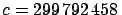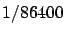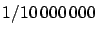Next: From anthropomorphic units to Up: Why does the meter Previous: Why does the meter

# Introduction

Presently, the unit of length in the International System (SI)  is a derived unit, related to the unit of time via the speed of light, assumed to be constant and of exact valuem/s.1 The unit of time is related to the period of a well defined electromagnetic wave.2The proportionality factors that relate conventional meter and second to more fundamental and reproducible quantities were chosen to make these units back compatible to the original units of the Metric System. In that system the second was defined asof the average solar day and the meter was related to the Earth meridian (namelyof the distance between the pole and the equator on the Earth's surface). Therefore, meter and second were initially not formally related to each other.

It is then curious to discover -- we say `discover' because this simple observation has surprised us, as well as many colleagues and friends -- that a simple pendulum of one meter in length `beats' the second, in the sense that each oscillation, i.e. half period, takes approximately one second.

A simple exercise (see Appendix A) shows that there is no deep physical connection between the two quantities. Other explanations are a mere, indeed remarkable numerical coincidence, or the fact that the `first meter' was already somehow a derived unit -- although never explicitly stated in official documents -- in the sense that particular fraction of that particular geometrical parameter of Earth was chosen to approximately match the length of the pendulum that beats the second.

In order to understand how believable the guess of an initially derived meter is, we have gone through the steps that led to the several proposals of a unit of length taken from nature. We have learned an interesting circular story of a unit of length that begins and ends with the second. At the beginning the unit of length was bound to the second by gravitational acceleration, taken at a reference point on the Earth's surface. Now the meter is bound to the second by the speed of light. In between (and somehow in parallel, at the very beginning) there have been the several attempts to define ``a unit of length that does not depend on any other quantity''3 : initially from the size of Earth; then from a conventional platinum bar; finally from the wavelength of a well defined electromagnetic radiation.

The recent relationship between the meter and the second is quite well known. Much less known is the fact that, just before the French Academy definition of the meter as a fraction of the Earth meridian, most scientists had considered the length of the pendulum that beats the second to be the most suitable unit of length. That consensus had steadily increased during the 18th century. However, in spring 1791 there was a metrological revolution inside the French Revolution. The result was a unit of length equal to a quadrant of the Earth's meridian (with practical unit a convenient decimal sub-multiple, namely).

Our interdisciplinary work wants to reason the plausibility of a meter initially bound to the second.Next: From anthropomorphic units to Up: Why does the meter Previous: Why does the meter
Giulio D'Agostini 2005-01-25